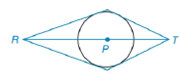Chapter 6.4, Problem 14E### Elementary Geometry for College St...

6th Edition
Daniel C. Alexander + 1 other
ISBN: 9781285195698

#### Solutions

Chapter
Section### Elementary Geometry for College St...

6th Edition
Daniel C. Alexander + 1 other
ISBN: 9781285195698
Textbook Problem
1 views

# Given circle P and points R − P − T such that R and T are in the exterior of circle P , suppose that tangents are constructed from R and T to form a quadrilateral (as shown). Identify the type of quadrilateral formeda) when R P > P T .b) when R P = P T .To determine

(a)

To identify:

The type of quadrilateral formed based on the condition when RP>PT.

Explanation

A quadrilateral in which two pairs of adjacent sides are equal and diagonals intersect at 90° and only one diagonal is bisected by the other is called a kite. Thus, in a kite AB=AD and BC=CD. Also, AEB=AED=BEC=DEC=90°.

The line that is perpendicular to the radius of a circle at its endpoint on the circle is a tangent to the circle. The tangents from an exterior point to the circle are two in numbers and the length of both is same at the point of intersection of the circle. In the figure below from point S two tangents are drawn touching the circle at R and T,

So, SR=ST

To determine

(b)

To identify:

The type of quadrilateral formed based on the condition when, RP=PT.

### Still sussing out bartleby?

Check out a sample textbook solution.

See a sample solution

#### The Solution to Your Study Problems

Bartleby provides explanations to thousands of textbook problems written by our experts, many with advanced degrees!

Get Started

#### Find more solutions based on key concepts